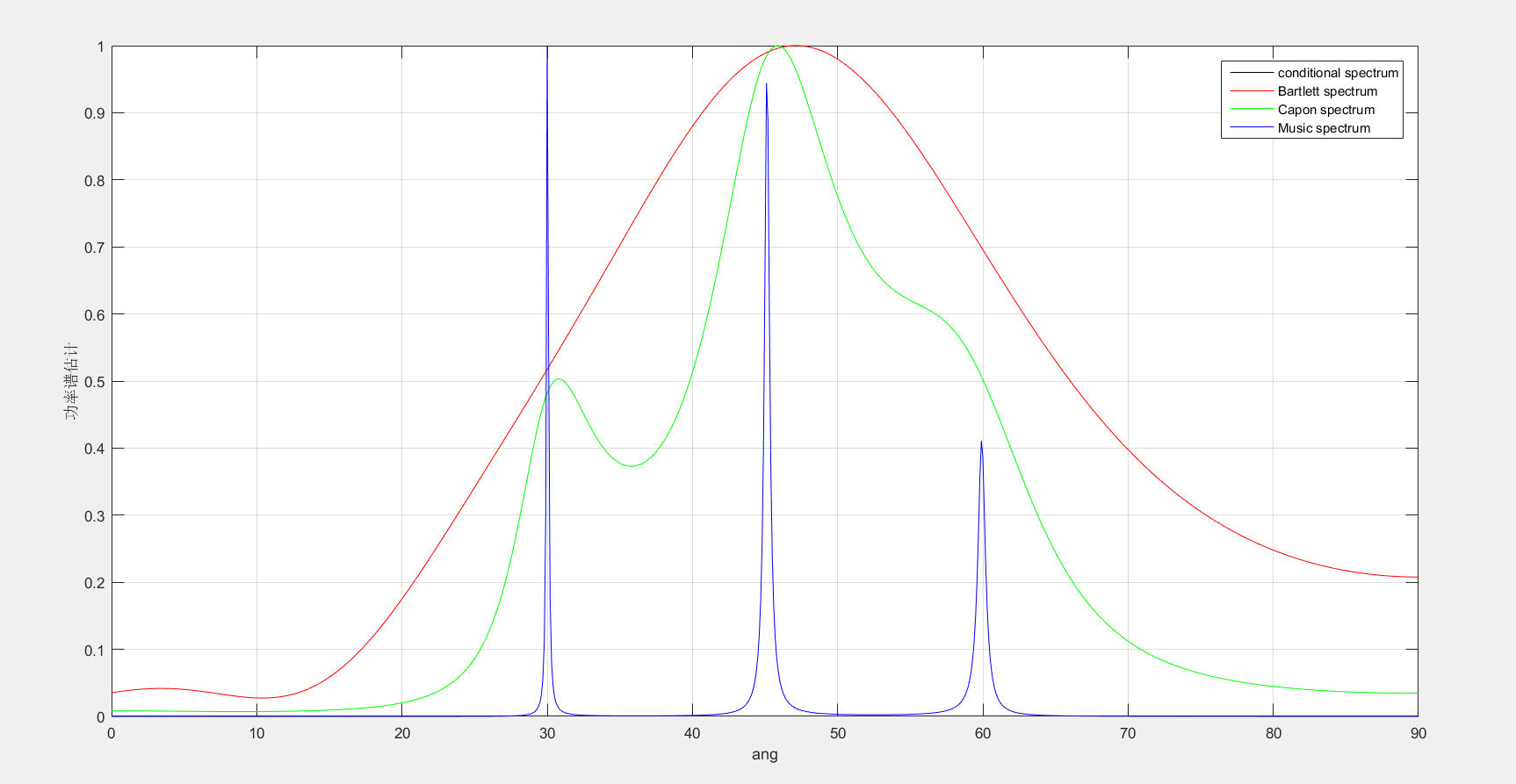• MUSIC算法 MATLAB代码 阵列信号处理
2021-11-20 22:18:54

MUSIC算法 MATLAB代码

J=sqrt(-1);
source_number=4;
source_doa=[30 45 60 135];
sensor_number=7;
snapshot_number=2000;
snr=10;

A=exp(-J*(0:sensor_number-1)'*pi*sin(source_doa*pi/180));
s=(randn(source_number,snapshot_number)+J*randn(source_number,snapshot_number))/sqrt(2);
x=A*s;
y=awgn(x,snr);
R=y*y'/snapshot_number;

[V,D]=eig(R);
Un=V(:,1:sensor_number-source_number);
Gn=Un*Un';

searching_doa=0:0.1:90;
for i=1:length(searching_doa)
a_theta=exp(-J*(0:sensor_number-1)'*pi*sin(pi*searching_doa(i)/180))
P_con(i)=abs(a_theta'*R*a_theta);
P_BF(i)=abs((a_theta'*R*a_theta)./(a_theta'*a_theta));
P_capon(i)=1./abs((a_theta'*inv(R)*a_theta));
P_music(i)=1./abs((a_theta'*Gn*a_theta));
end
plot(searching_doa,P_con/max(P_con),'k');hold on;
plot(searching_doa,P_BF/max(P_BF),'r'); hold on;
plot(searching_doa,P_capon/max(P_capon),'g'); hold on;
plot(searching_doa,P_music/max(P_music),'b'); hold off;grid on;
xlabel('ang');
ylabel('功率谱估计');
legend('conditional spectrum','Bartlett spectrum','Capon spectrum','Music spectrum');人人为我，我为人人
记得点个赞啊python matlab https
更多相关内容
• 利用MATLAB实现了二维MUSIC算法，估计波达方向的俯仰角和方位角，给出归一化空间谱，对学习二维MUSIC算法有一定的帮助
• 针对目前多信号环境下二维DOA参数测量问题，对二维MUSIC算法分维处理中的参数配对耦合问题进行了分析，得出了耦合阵列结构的特征，即二维MUSIC算法分维处理中，耦合的产生与否由阵列的横坐标矢量与纵坐标矢量的线性...
• 阵列信号处理，多重信号分类，music求根music，二维music
• 二维ROOT-MUSIC DOA估计算法的MATLAB实现代码，可以参考学习。
• 针对信源数目过估计下二维MUSIC算法会出现虚假信号和欠估计下某些谱峰消失的问题，通过将降维技术与一维噪声子空间算法相结合，提出一种基于正交阵列结构的修正二维MUSIC算法。该算法将复杂的二维处理问题转化为3个...
• 得到了3种算法的角度RMSE的对比分析，可知在同样仿真条件下，二维增广矩阵束算法最优，二维MUSIC算法次之，二维干涉算法最差。同时，构建了相应的试验场景，通过试验分析上述二维谱估计算法的空间探测性能，得到的...阵列流型
• 均匀线阵下的music算法，经典music算法，高斯白噪声下的窄带信号，运行无误，希望对广大学者有所帮助。
• 想问下二维music的时延和多普勒估计怎么写代码，是在导向矢量上变化吗？
• MUSIC算法MATLAB仿真程序 源代码 MUSIC算法MATLAB仿真程序，挺有用的
• 几种music-DOA估计算法的性能比较（RMSE对比）
• 音乐湖 PC 端 由于收到网易云音乐和 QQ 音乐的警告函，登录/云歌单服务已关闭，可继续使用离线歌单，有服务器资源可自行搭建云歌单，所有代码均已开源。打包介绍见build.md 简介 歌曲 Api 涵盖网易云、QQ 音乐...electron vue
• Music player and server for ownCloud and Nextcloud. Shows audio files stored in your cloud categorized by artists and albums. Supports mp3, and depending on the browser, many other audio formats too. ...
• 2、主要实现了 二维MUSIC估计算法下，互质面阵的模糊解决算法。 【代码特点】：参数化编程（参数可方便修改）、思路步骤清晰、注释明细。 【适合对象】：信号处理、雷达专业学生。 【乱码问题】：文件打开如果出现...
• 南京航空航天大学张小飞经典MUSIC算法可直接用！DOA估计的入门搭配教材更好！
• MUSIC算法的MATLAB实现程序 源代码（2017年11月7日） 现代功率谱的空间谱估计法MUSIC算法，没调用MATLAB中现成函数，自己编程实现的。
• 针对经典二维多重信号分类(Multiple Signal Classification,MUSIC)算法在低信噪比和小快拍数情况下,分辨率受阵列孔径限制的问题,提出了一种改进的基于MUSIC算法二维测向算法.该方法利用MUSIC谱函数极大值点处对...
• MUSIC算法是经典的超分辨波达方向估计算法，受到广大学者的深入研究，该算法利用信号子空间与噪声子空间的正交性完成波达方向估计，具有重要的研究价值
• ## MUSIC算法推导及代码实现

万次阅读 多人点赞 2019-05-13 18:14:57
MUSIC算法是一种基于子空间分解的算法，它利用信号子空间和噪声子空间的正交性，构建空间谱函数，通过谱峰搜索，估计信号的参数。对于声源定位来说，需要估计信号的DOA。MUSIC算法对DOA的估计有很高的分辨率，且对...

### 简介

MUSIC (Multiple Signal Classification)算法，即多信号分类算法，由Schmidt等人于1979年提出。MUSIC算法是一种基于子空间分解的算法，它利用信号子空间和噪声子空间的正交性，构建空间谱函数，通过谱峰搜索，估计信号的参数。对于声源定位来说，需要估计信号的DOA。MUSIC算法对DOA的估计有很高的分辨率，且对麦克风阵列的形状没有特殊要求，因此应用十分广泛。

### 原理

MUSIC算法是基于远场窄带信号的子空间算法，经典的远场窄带DOA估计的阵列信号模型如图1，

1. 数学模型

远场窄带DOA估计数学模型为，

其中，为采样点数，为M个阵元的输出，为方向响应向量，P为信号源的数量，为入射信号，为阵列噪声。

2. 计算协方差矩阵

由于各阵元的噪声互不相关，也不和信号相关，因此X(n)的协方差矩阵为，

其中，表示入射信号的协方差矩阵，操作为复共轭转置。

实际上，采集的接收数据矩阵是有限长的，需要对有限的采样数据的协方差矩阵进行最大似然估计，

3. 进行特征值分解

对该协方差矩阵进行特征值分解，对分解得到的特征值进行大小排列，把与信号源数量P相等的最大的P个特征值所对应的特征向量组成的特征空间，称为信号子空间，即；把剩下的(M-P)个特征值对应的特征向量组成的特征空间称为噪声子空间，即,

4. 构建空间谱

空间谱计算公式如下，

进行谱峰搜索个极大值对应的就是信号源的方向。

### 实验仿真

对于一维均匀线性阵列（ULA，Uniform Linear Array），每个阵元等间距线性排列，其MUSIC算法如下：

clear; close all;
%%%%%%%% MUSIC for Uniform Linear Array%%%%%%%%
N = 8;               % 阵元个数
M = 3;               % 信源数目
theta = [-30 0 60];  % 待估计角度
snr = 10;            % 信噪比
K = 512;             % 快拍数

dd = 0.5;            % 阵元间距
d=0:dd:(N-1)*dd;
% 构建信号模型
S=randn(M,K); X=A*S;
X1=awgn(X,snr,'measured');
% 计算协方差矩阵
Rxx=X1*X1'/K;
% 特征值分解
[EV,D]=eig(Rxx);
EVA=diag(D)';
[EVA,I]=sort(EVA);
EV=fliplr(EV(:,I));
En=EV(:,M+1:N);    % 信号子空间

% 遍历每个角度，计算空间谱
for iang = 1:361
angle(iang)=(iang-181)/2;
a=exp(-1i*2*pi*d*sin(phim)).';
Pmusic(iang)=1/(a'*En*En'*a);
end
Pmusic=abs(Pmusic);
Pmusic=10*log10(Pmusic);
h=plot(angle,Pmusic);
set(h,'Linewidth',0.5);
xlabel('入射角/(degree)');
ylabel('空间谱/(dB)');
set(gca, 'XTick',[-90:30:90]);
grid on;

对于二维均匀圆阵（UCA，Uniform Circle Array），每个阵元等间角度的分布在半径为r的圆周上。其MUSIC算法如下：

clear all; close all;
%%%%%%%% MUSIC for Uniform Circle Array%%%%%%%%
r=1;                                            % 半径(m)
N=16;                                           % 阵元数目
d=2*r*sin(pi/N);

M=1;                                            % 信源数量
gamma=2*pi/N*(0:N-1);                           % 和参考阵元的角度
fc=16000;                                       % 采样率
c=340;                                          % 声音的速度（m/s）
lambda=c/fc;

a_theta=45;                                     % 仰角
a_phi=90;                                       % 方位角

zeta=2*pi/lambda*r*sin(a_theta*pi/180);
A=exp(1i*zeta*cos((a_phi-gamma)*pi/180)).';     % 导向矢量

K=100;                                          % 快拍数
t=(0:K-1)/1000;

% 构建信号模型
S=sin(2*pi*fc*t);
X=A*S;
snr=10;
X1=awgn(X,snr,'measured');
% 计算协方差矩阵
R=X1*X1'/K;
% 特征值分解
[Q,D]=eig(R);
[D,I]=sort(diag(D),1,'descend');
Q=Q(:,I);
Qn=Q(:,M+1:N);                                  % 信号子空间

theta=0:90;
phi=0:1:360;

p_MUSIC=zeros(length(theta),length(phi));

for ii=1:length(theta)
for iii=1:length(phi)
zeta=2*pi/lambda*r*sin(theta(ii)*pi/180);
A=exp(1i*zeta*cos((phi(iii)-gamma)*pi/180)).';
p_MUSIC(ii,iii)=(1/(A'*(Qn*Qn')*A));
end
end

mesh(phi,theta,abs(p_MUSIC))
grid on;xlabel('仰角');ylabel('方位角');zlabel('PMUSIC');title('UCA MUSIC');

展开全文• This is for DOA estimation,and its easier than traditional MUSIC algrithomlin...